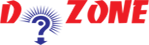﻿ Python Program for Multiplication Table## Python Program To Display the Multiplication Table

Through this program, you can easily make the multiplication table of variable numbers ranges from 1 to 10. To explain this program, we have used the for loop python programming to display the multiplication table of 11.

``````
Python Code
''' Python program to find the
multiplication table (from 1 to 10)'''

num = 11

# To take input from the user
# num = int(input("Display multiplication table of? "))

# use for loop to iterate 10 times
for i in range(1, 11):
print(num,'x',i,'=',num*i)
Output
11 x 1 = 11
11 x 2 = 22
11 x 3 = 33
11 x 4 = 44
11 x 5 = 55
11 x 6 = 66
11 x 7 = 77
11 x 8 = 88
11 x 9 = 99
11 x 10 = 110

```
```

Note: To repeat it 10 times, you need to use for loop as well as the range() function is used. Now, enter two arguments inside range function like (1,11). The first number must be greater to 1 and the second one should be less than 11 ie. 10.

So learners see the tutorial and try it by changing the values of num in the program.

## Other Python Programs

PythonTraining, 258-FF , Katewa Nagar, New Sanganer Rd, Jaipur, 302019